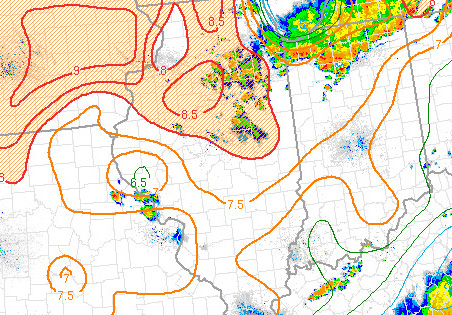[--MAIN HOME--] [--ALL HABYHINTS--] [--FACEBOOK PAGE--]

 GRAPHICAL AREA DISPLAY: 850 to 500 mb LAPSE RATE

METEOROLOGIST JEFF HABY

A lapse rate is a change in temperature with height. This value can be used to infer instability. The higher the value then the higher the instability. Values less than 6 are generally stable while values of 7.5 or above are very unstable. Values between 6 and 7.5 generally mean there is instability and it can be released if there is adequate dynamic lifting to lift a parcel into an unstable elevation in the troposphere. The same holds for values of 7.5 and above, but the convection is more likely to be explosive if instability release occurs at these high values.

The 850 and 500 mb lapse rate has units of “C/km”. The “C” value is found by taking the temperature difference between 850 and 500 mb. The “km” value is the vertical distance between the 850 and 500 mb pressure surfaces. For example, if the temperature is 25 C at 850 mb, the temperature is -10 C at 500 mb, and the distance from 850 to 500 mb is 4.2 km, then the lapse rate is (25 –(-10) / 4.2 = 35/4.2 = “8.3 C/km”. For higher elevation regions, such as locations where the 850 mb level is near or below the ground surface, then the 700 to 500 mb lapse rate is used in order to represent the lapse rate.

The image below shows an example of a lapse rate chart. The values are on the high side, thus thunderstorms that develop should have strong updrafts. This increases the potential for severe weather.